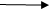Sizeof() operator in C
Ratings:
(4)
Views:837## size of : -

It is a operator can keyword in ‘c’ language

• it is a urinary operator which returns size of even argument.
• size of operator always returns unsigned integer value only and which value is greater than “Zero”

### void main()

{ int i; char ch; float f; printf("\n size of int %d”, sizeof(i)); printf(“\n size of char: %d ”, sizeof ch); printf(“\n size of float : %d”, size of F); } o/p: size of int : 2 size of char:1 size of float:4   sizeof(int)2 bytes sizeof(signed)2 bytes sizeof(short)2 sizeof(long)4 sizeof(25)2 sizeof(40000u)2 sizeof(655361)4 sizeof(char)1 sizeof(‘A’)2              // sizeof(65) --- >2 char ch; ch=’A’; sizeof(ch)1 size of character or character variable is 1 byte but the size of character constant is 2 bytes, because by default character constant returns integer value. i.e ‘ASCII’  value of a character. So, we will get 2 bytes sizeof(ch)1 sizeof(float)4 sizeof(double)8 sizeof(long double)  -10 sizeof(short double)error sizeof(12.8)8 //double sizeof(12.8f)4   //float sizeof(12.8L)10    // long double sizeof(3.0)8 sizeof(3.5)8

### void main()

{ int i; i=0; printf(“\nsize1:%d”, size(i)); printf(“\nsize2:%d”, size(i*1 5l)); printf(“\nsize3:%d”, size(i/2.0)); printf(“\nsize4:%d”, size(i/2.0f)); printf(“\nsize5:%d”, size(i=i*15)); printf(“\ni=%d”,i); o/p : size1         :        2       //int Size2    :        4       // int,longlong size3     :        8       //int,doubledouble size4     :        4       //int, floatfloat size 5     :        2       //int,intint i=0   in size of operator all expressions can be evaluated except assignment.

### void main()

{ int a; a=10; printf(“\n size of a: %d”, sizeof(++a));     //a=a+1   ignore inclure printf(“/n a=%d”,a); } o/p : size of a:2 a=10